# Objects and Polygonal Boundaries

In some of my recent perusal of image processing questions on MATLAB Answers, I have come across several questions involving binary image objects and polygonal boundaries, like this.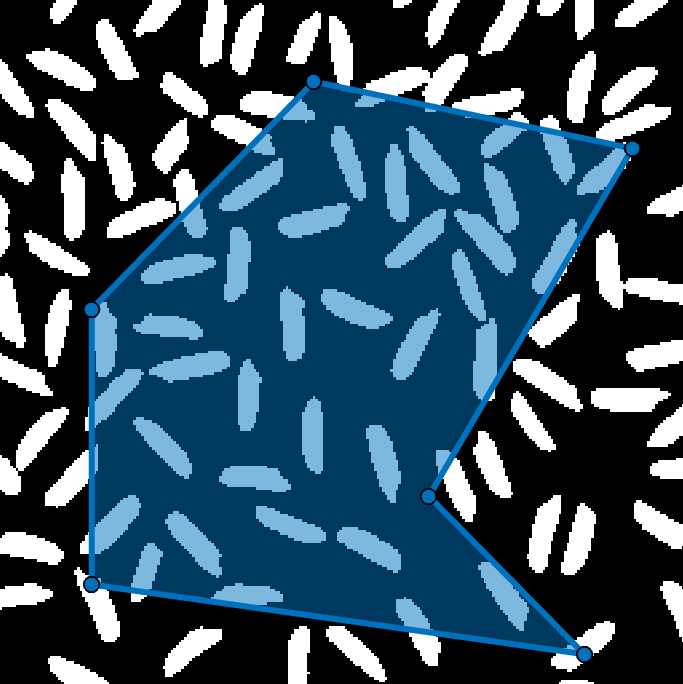The questions vary but are similar:
• How can I determine which objects touch the polygon boundary?
• How can I get rid of objects outside the boundary?
• How can I get rid of objects outside or touching the boundary?
• How can I get rid of objects within a certain distance of the boundary?
Today I want to show you how to answer all these questions and more besides, using these fundamental operations:
(Or, as an alternative to drawpolygon, images.roi.Polygon, and createMask, you can use roipoly and poly2mask.)
Here is the image I'll be using today:
image_url = "https://blogs.mathworks.com/steve/files/rice-bw-1.png";
imshow(A)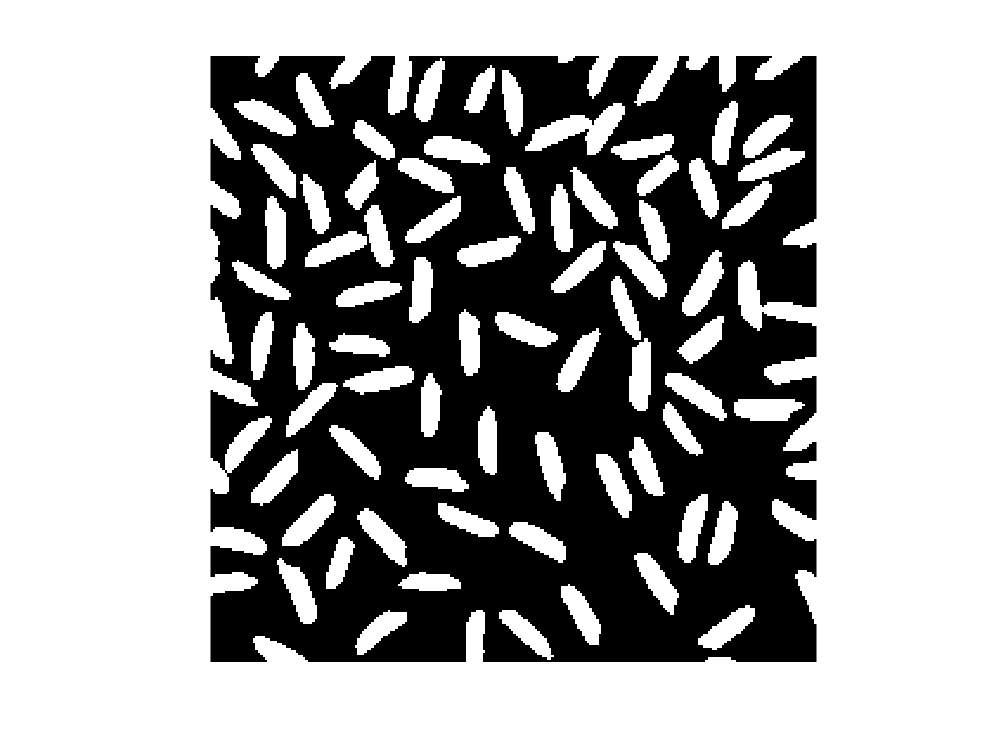And here is my polygon.
xy = [ ...
118 31
35 116
35 219
219 245
161 186
237 56];
I'll be drawing it using a helper function, showpoly, which is at the end of this post. The helper function uses drawpolygon, like this:
imshow(A)
p = drawpolygon(Position = xy, FaceAlpha = 0.5)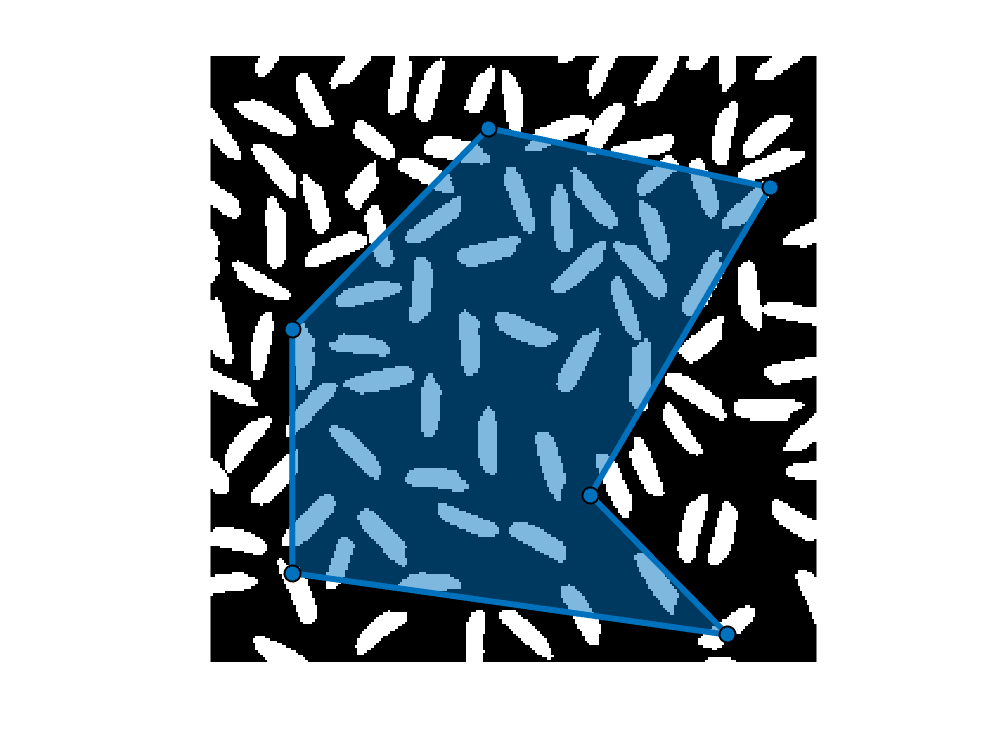p =
Polygon with properties: Position: [6×2 double] Label: '' Color: [0 0.4470 0.7410] Parent: [1×1 Axes] Visible: on Selected: 0 Show all properties
Note that drawpolygon creates an images.roi.Polygon (or just Polygon). The Polygon object is one of a variety of region-of-interest (ROI) objects in the Image Processing Toolbox. You can customize many aspects of its appearance and behavior, and it is useful for implementing apps that work with ROIs.
Of particular interest for today's topic is the Polygon function called createMask. This function returns a binary image with white pixels inside the polygon.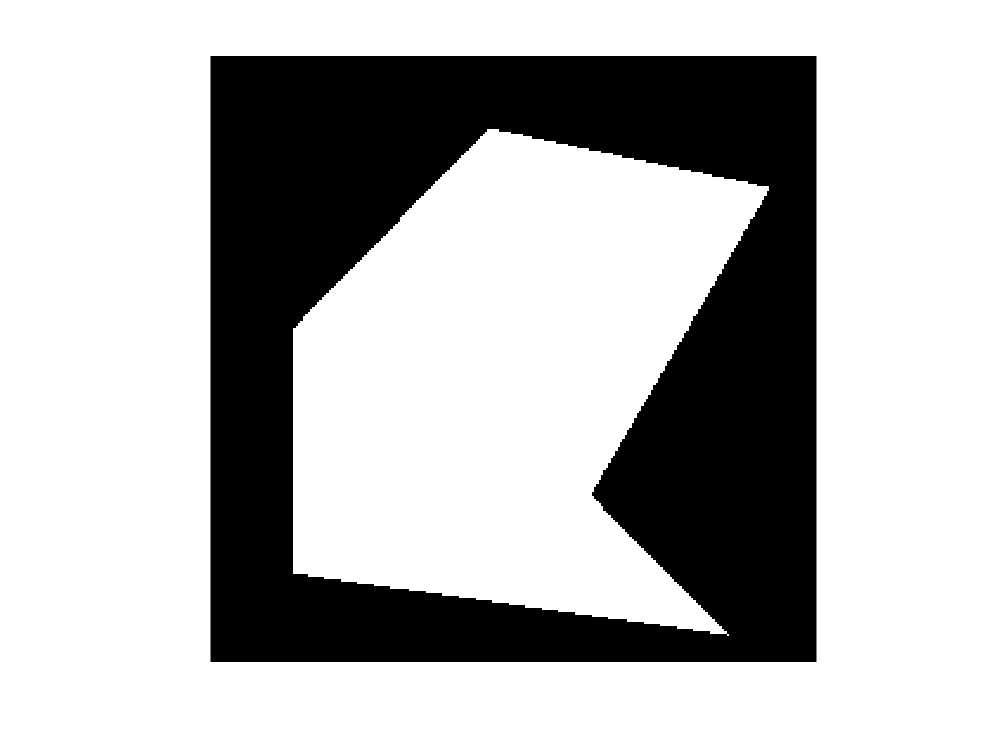## Objects completely or partially inside the polygon

Now we can start answering the object-polygon questions. First up: which objects are inside the polygon, either completely or partially?
One of the things that makes MATLAB fun for image processing is using logical operators with binary images. Below, I compute the logical AND of the original binary image with the polygon mask image.
imshow(B)
showpoly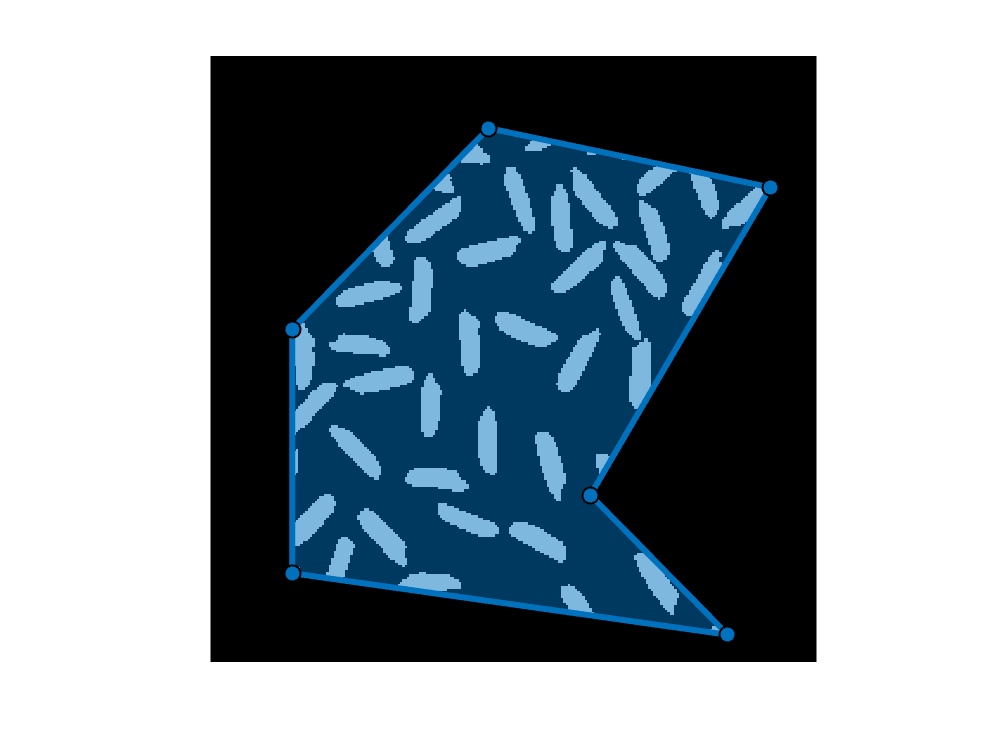Next, I apply morphological reconstruction using imreconstruct. This function takes a marker image and expands its objects outward, while constraining the expansion to include only object pixels in the second input. This is a convenient way to convert partial objects back into their full, original shapes. The result, C, is an image containing objects that are completely or partially within the polygon.
C = imreconstruct(B,A);
imshow(C)
showpoly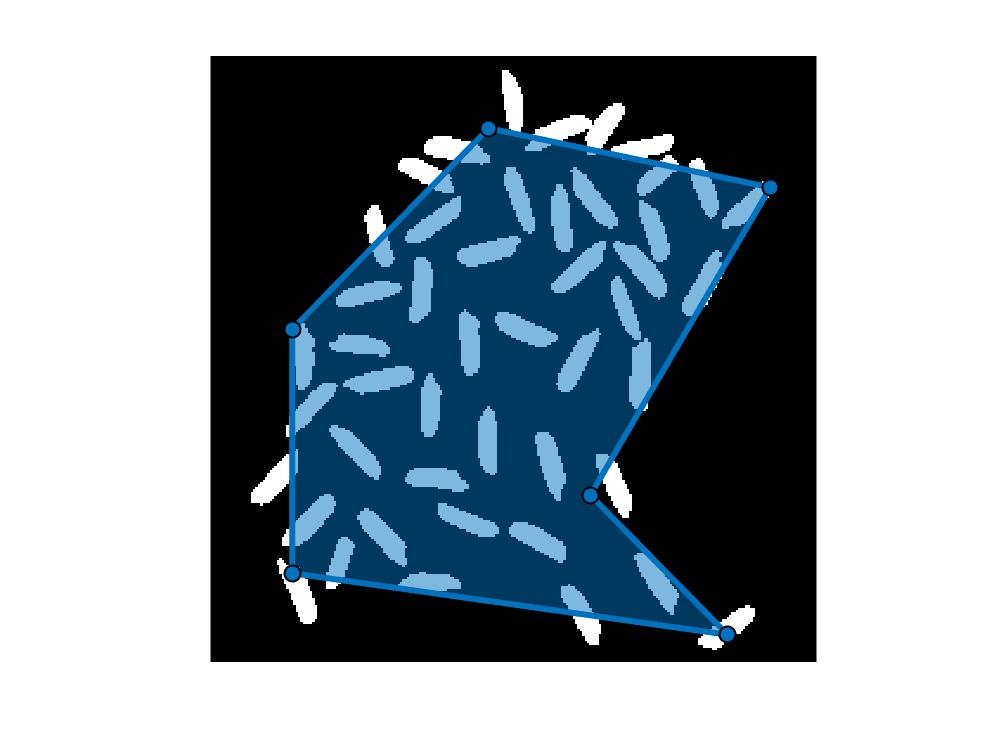## Objects touching the perimeter of the polygon

Next question: which objects touch the edge, or perimeter, of the polygon? I'll start with bwperim on the polygon mask to compute a perimeter mask.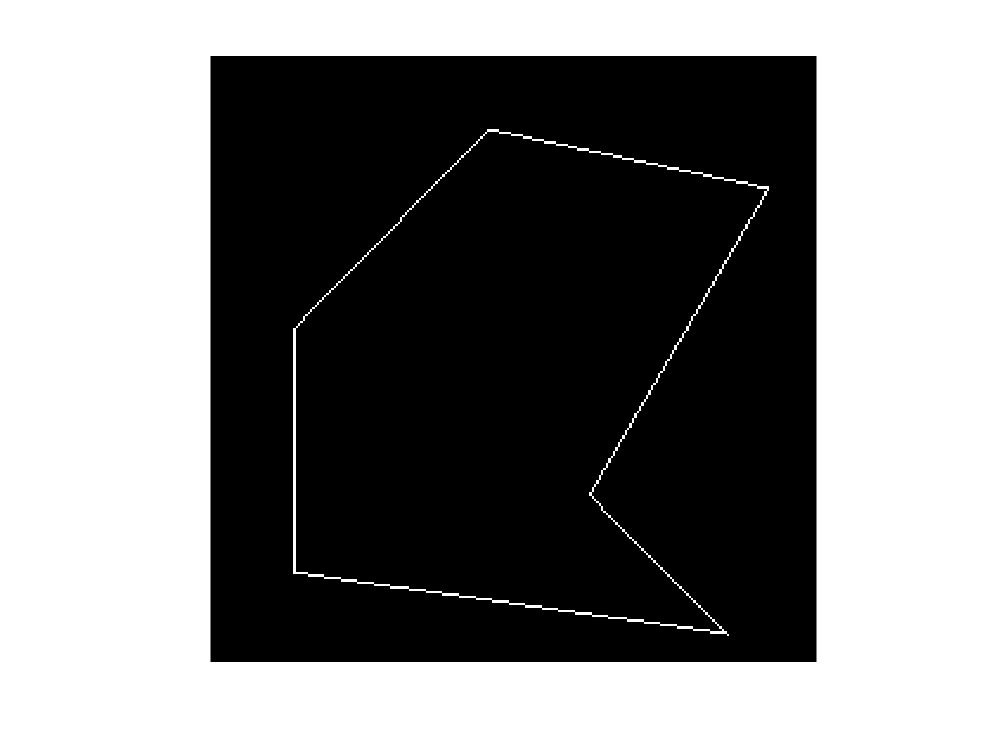Going back to the logical & operator gives us the perimeter pixels that are part of any of the objects.
imshow(D)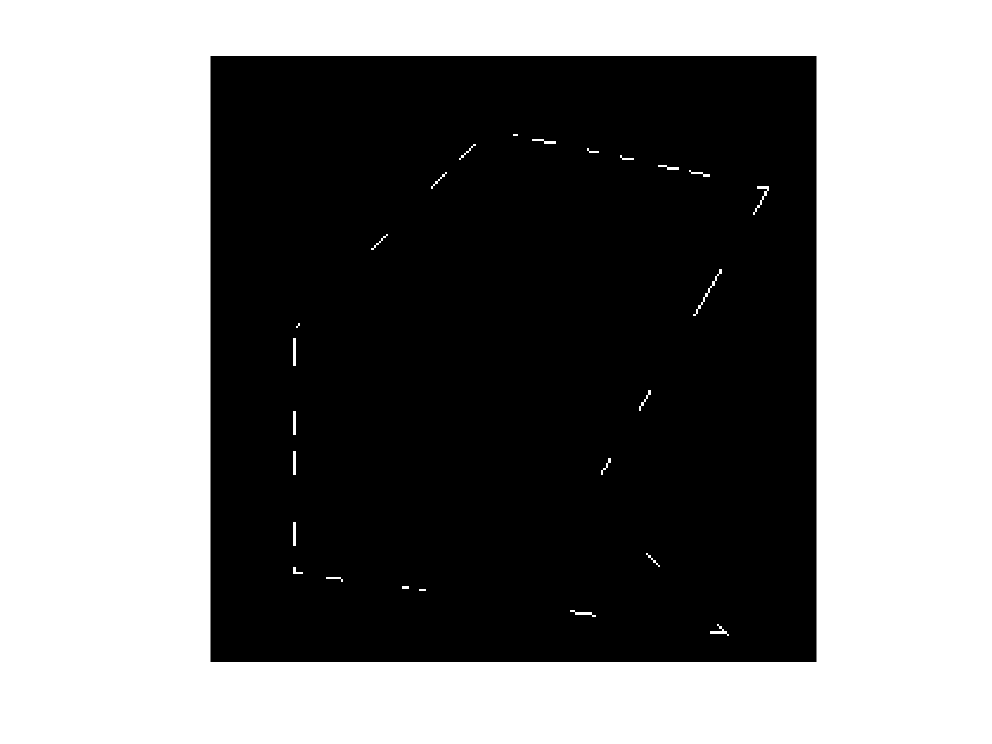Morphological reconstruct is useful again here, to "reconstruct" the full shapes of objects from the object pixels that lie along the polygon perimeter.
J = imreconstruct(D,A);
imshow(J)
showpoly();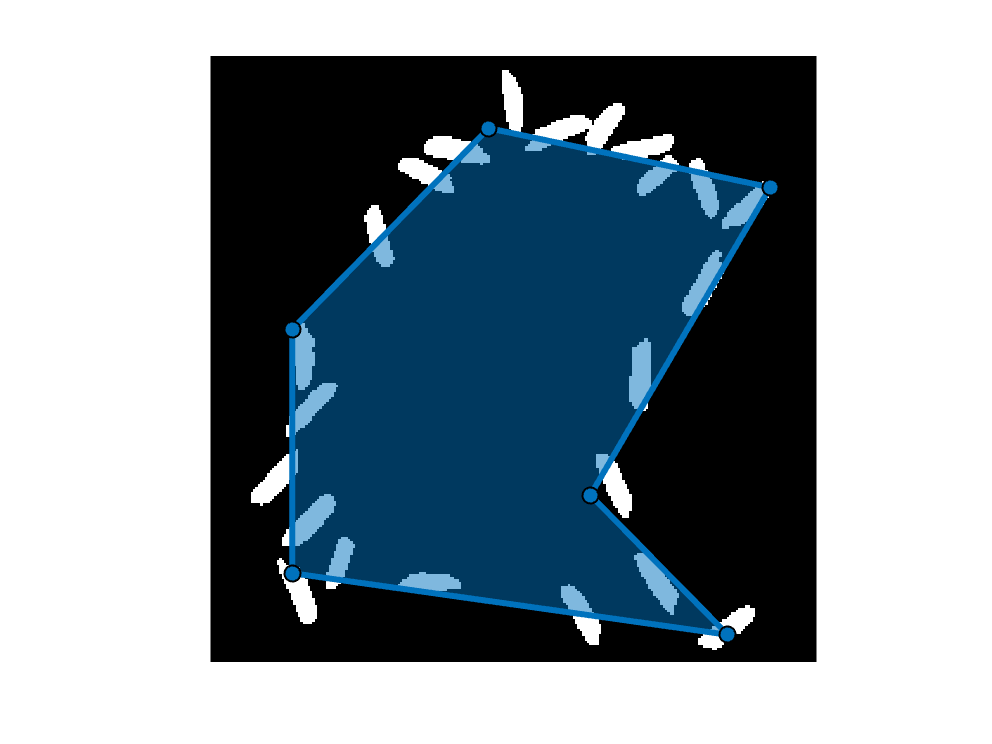## Objects outside the polygon

Third question: which objects lie completely outside the polygon? Recall that image C, computed above, is the objects that are completely or partially inside the polygon. The objects that we want, then, are the objects that are in A but not in C. You can compute this using A & ~C or setdiff(A,C).
objects_outside = A & ~C;
imshow(objects_outside)
showpoly();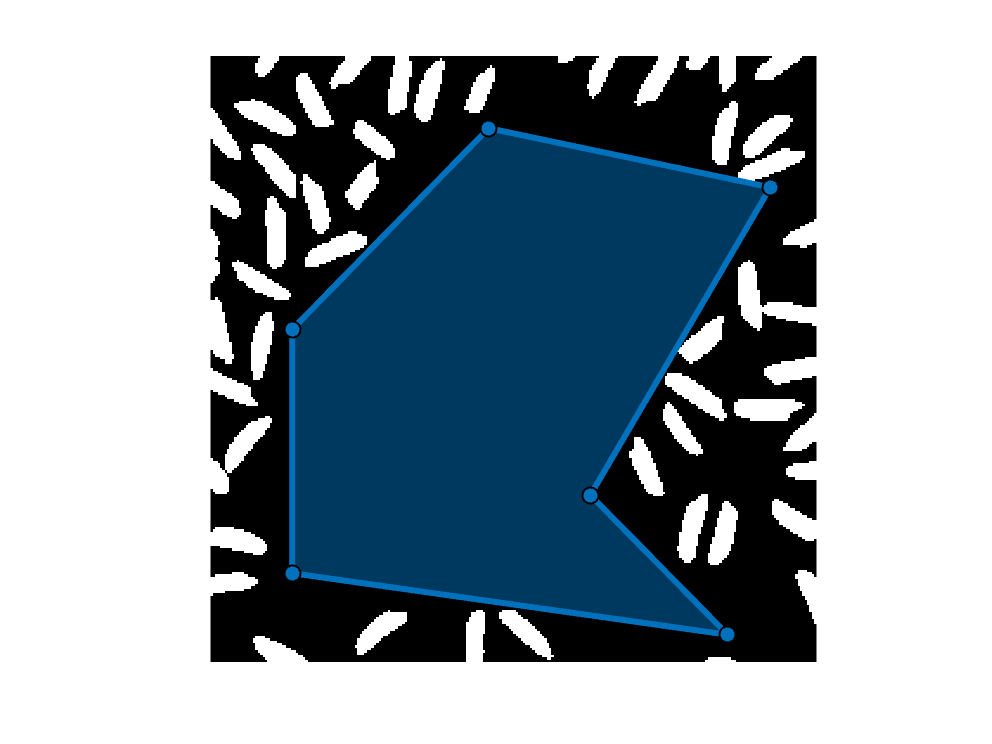What about the object near the upper right polygon vertex? Is it really completely outside the polygon?
axis([215 230 45 60])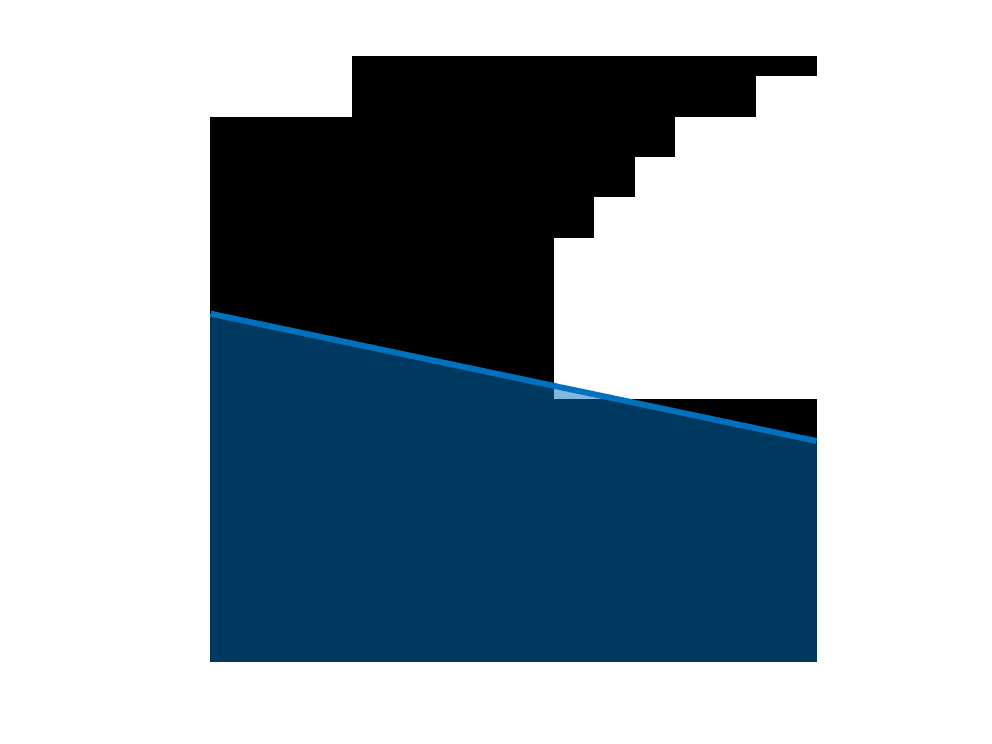It looks like a sliver of white is inside the polygon. Why is that?
I'll explain by showing the polygon mask, the outside objects, the polygon itself, and the pixel grid. I'll use imshowpair to both the polygon mask and the outside-objects mask. (The function pixelgrid is on the File Exchange.)
figure
showpoly();
axis([215 230 45 60])
pixelgrid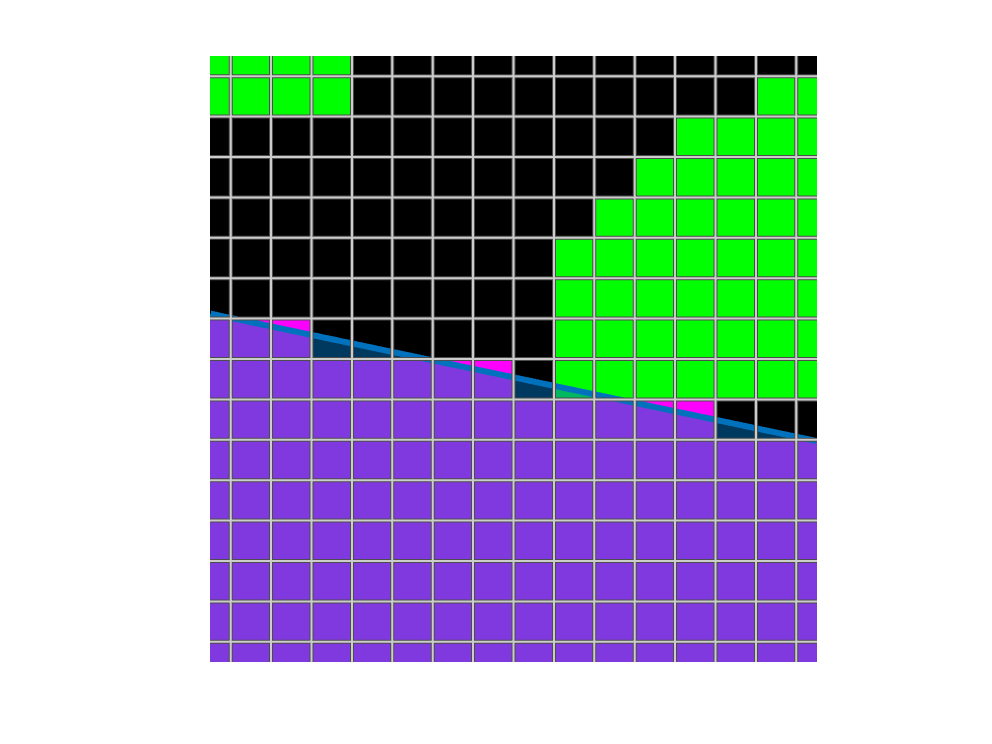When a polygon only partially covers a pixel, the createMask has certain tie-breaking rules to determine whether a pixel is inside or outside. You can see the tie-breaking rules play out in several pixels above.

## Objects inside and at least 20 pixels away the polygon boundary

The fourth question is which objects are not only inside the polygon, but at least 20 pixels away from the polygon boundary? To answer this question, I'll bring in another tool: the distance transform, as computed by bwdist.
First, though, let's start by applying the logical NOT (or complement) operator, ~. Applying it to the polygon mask image gives us a mask showing the pixels that are outside the polygon. I'll turn the axis box on so you can see the extent of the exterior mask.
axis on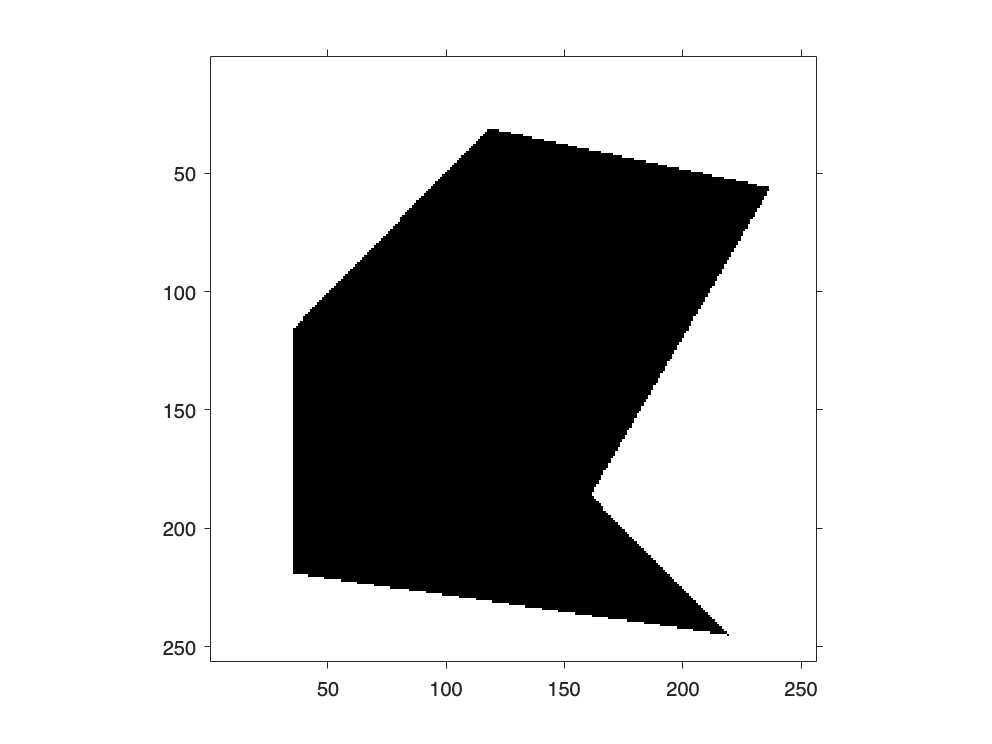The distance transform computes, for every image pixel, the distance between that pixel and the nearest foreground pixel. If a pixel is itself a foreground pixel, then the distance transform at that pixel is 0. By computing the distance transform of the exterior mask, we can find out how far every pixel is from the closest pixel in the polygon exterior.
(Alternative approach: consider the buffer method of polyshape object.)
When displaying a distance transform as an image, the autoranging syntax of imshow is useful. Just specify [] as the second argument when displaying intensity values, and the minimum value will get automatically scaled to black, and the maximum intensity value will get automatically scaled to white.
imshow(G,[])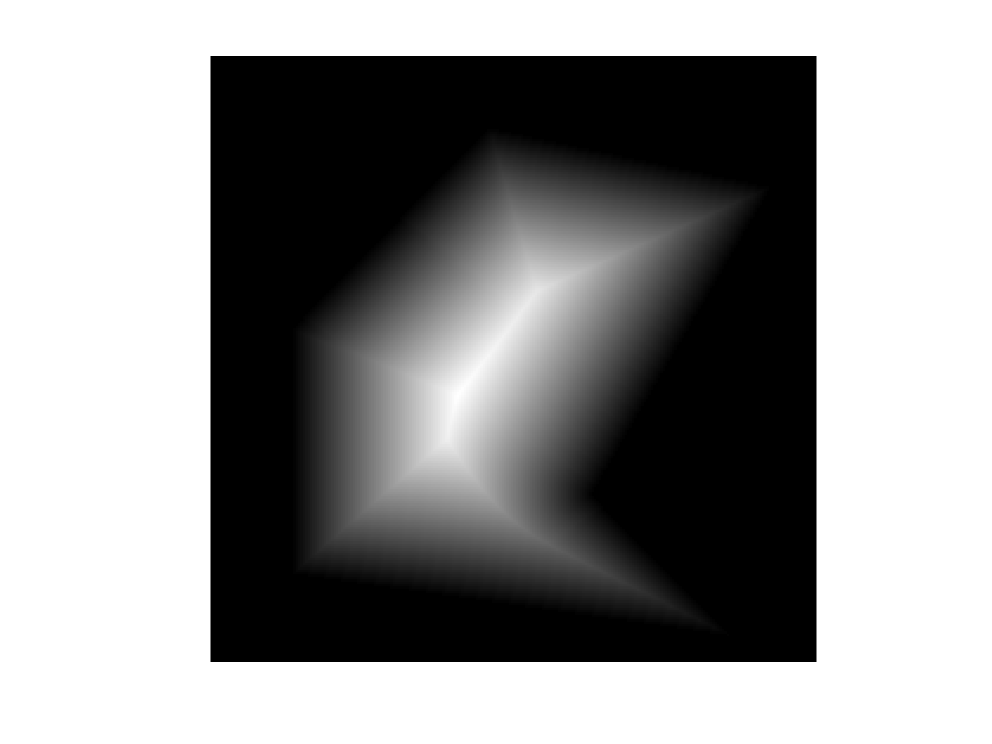Now let's compute a mask of pixels at least 20 pixel units away from the exterior mask by using a relational operator, >=.
showpoly();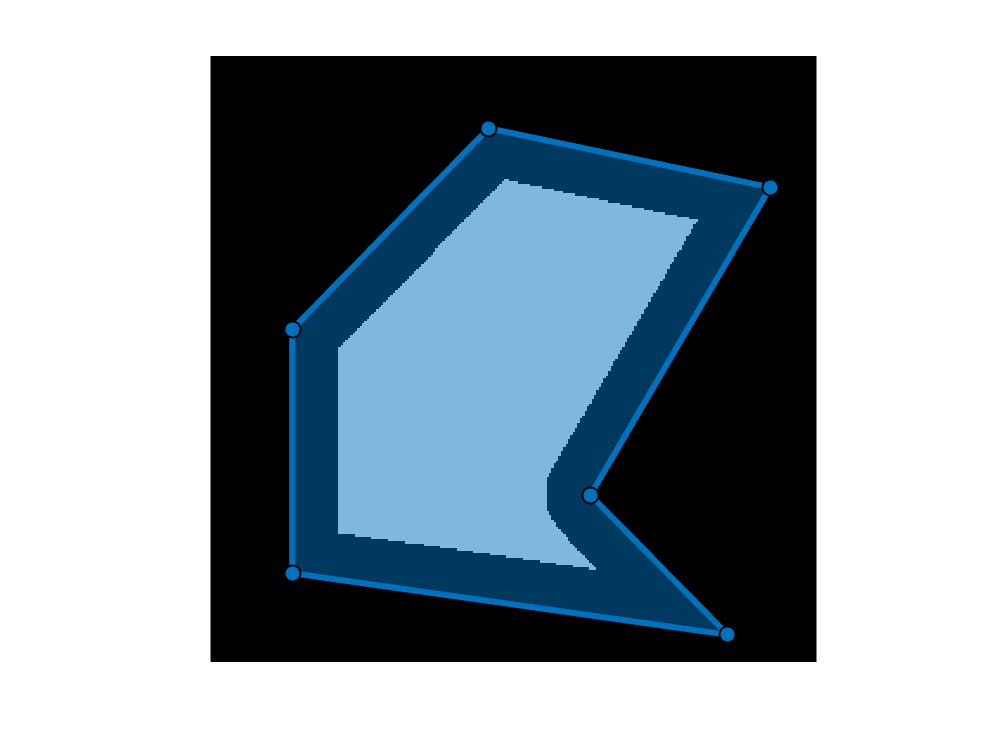Using the techniques described above, compute an image containing the objects that are completely outside the interior buffered mask.
H = imreconstruct(A & ~interior_mask_with_buffer, A);
imshow(H)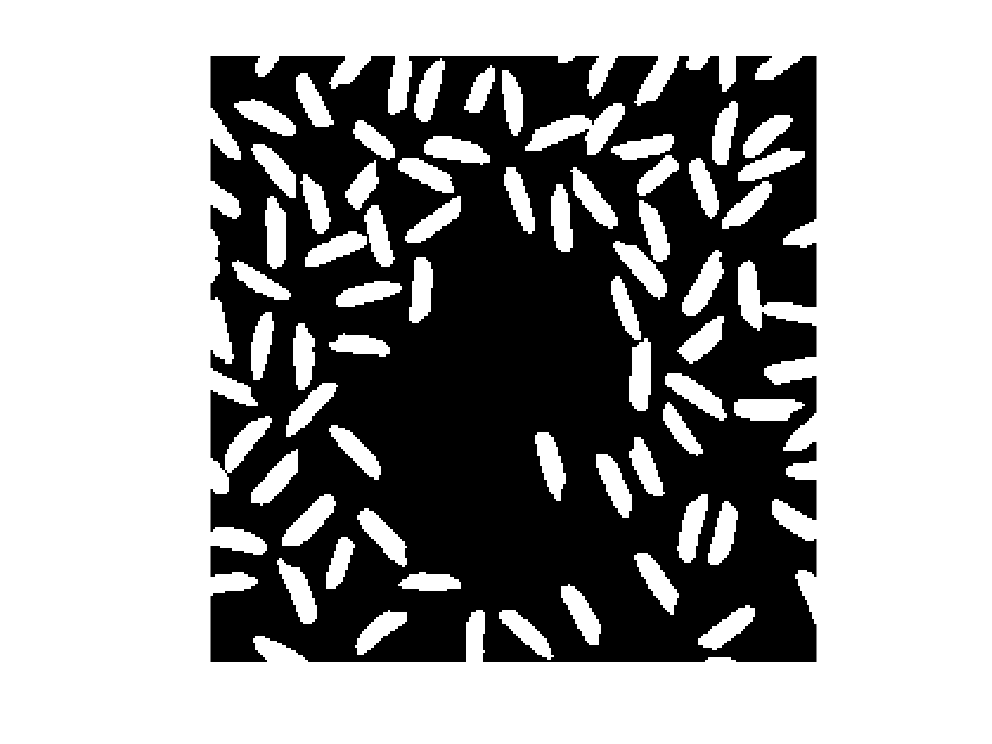Next, find the objects that are in A but not in H.
I = A & ~H;
showpoly();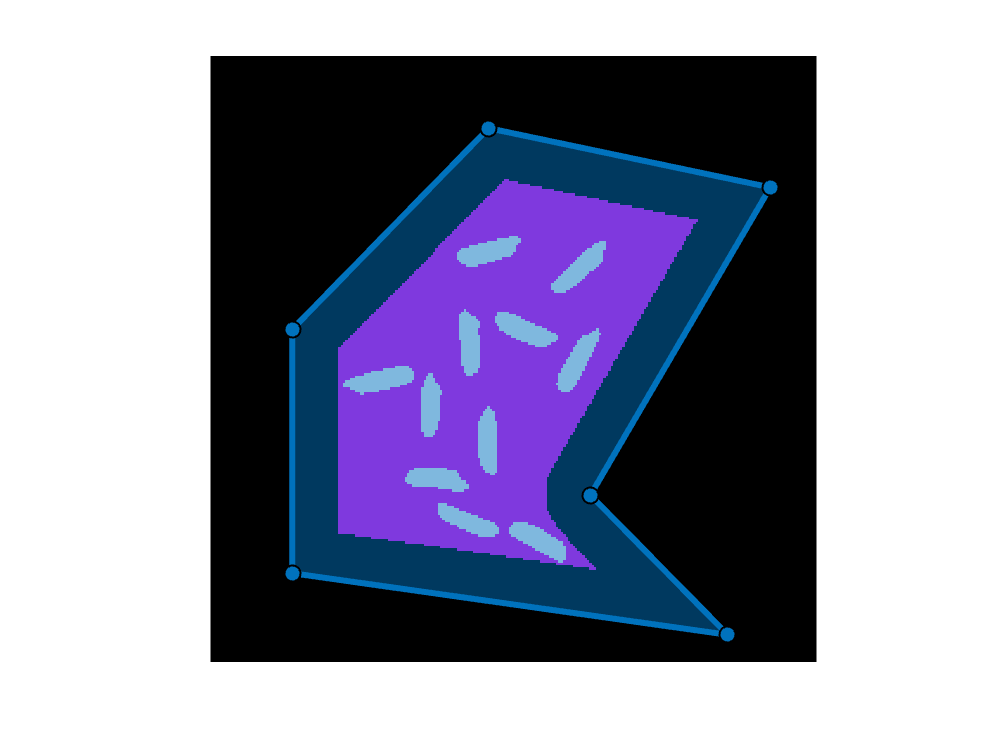## Objects not within 20 pixels of the polygon border

The final question for today is which objects are not within 20 pixels of the polygon border. Start by computing the distance transform of the perimeter mask.
imshow(J,[])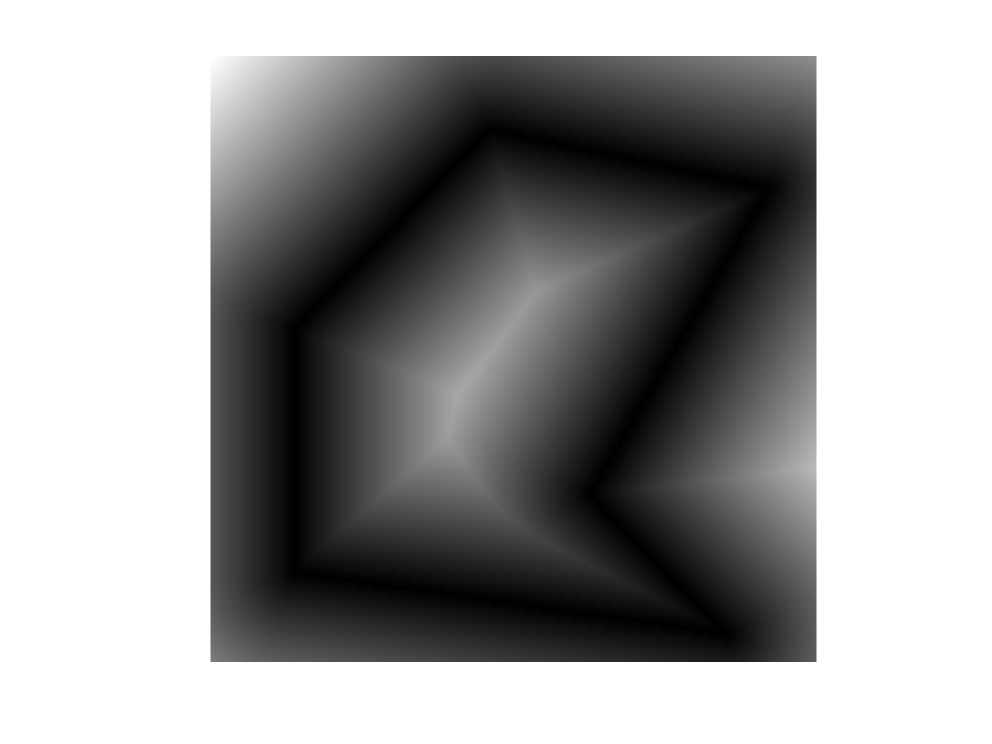Use a relational operator to compute a buffered perimeter mask.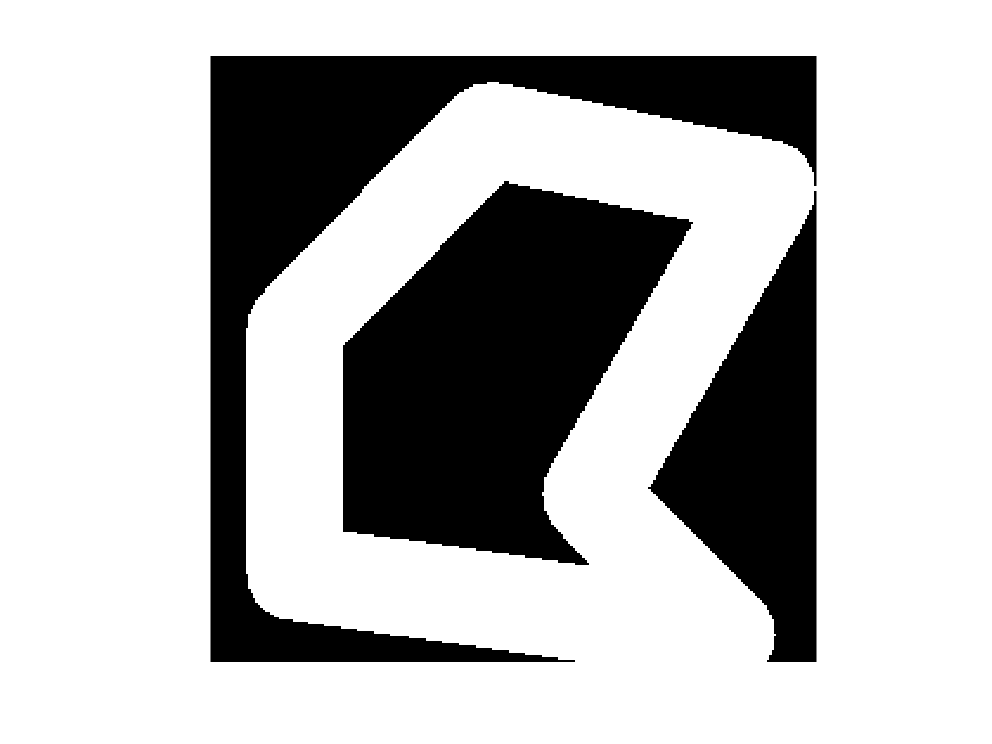Use the techniques described above to find all of the objects that are competely or partially within the buffered perimeter mask region.
L = imreconstruct(K,A);
imshow(L)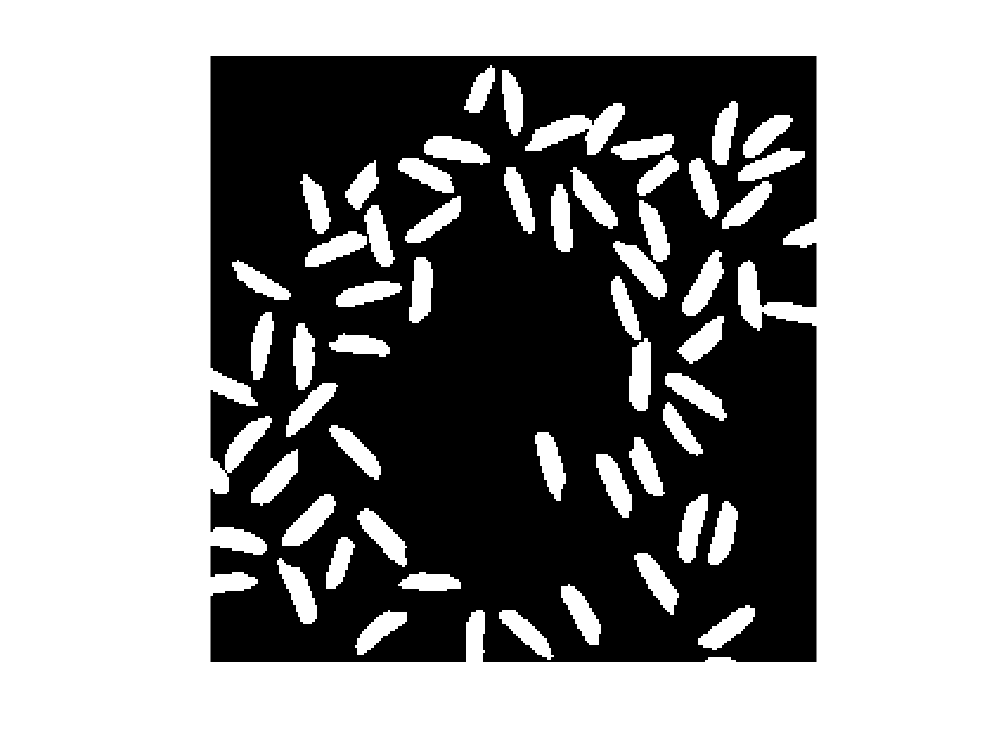The final answer is the objects that are in the original image, A, but not in L.
M = A & ~L;
showpoly();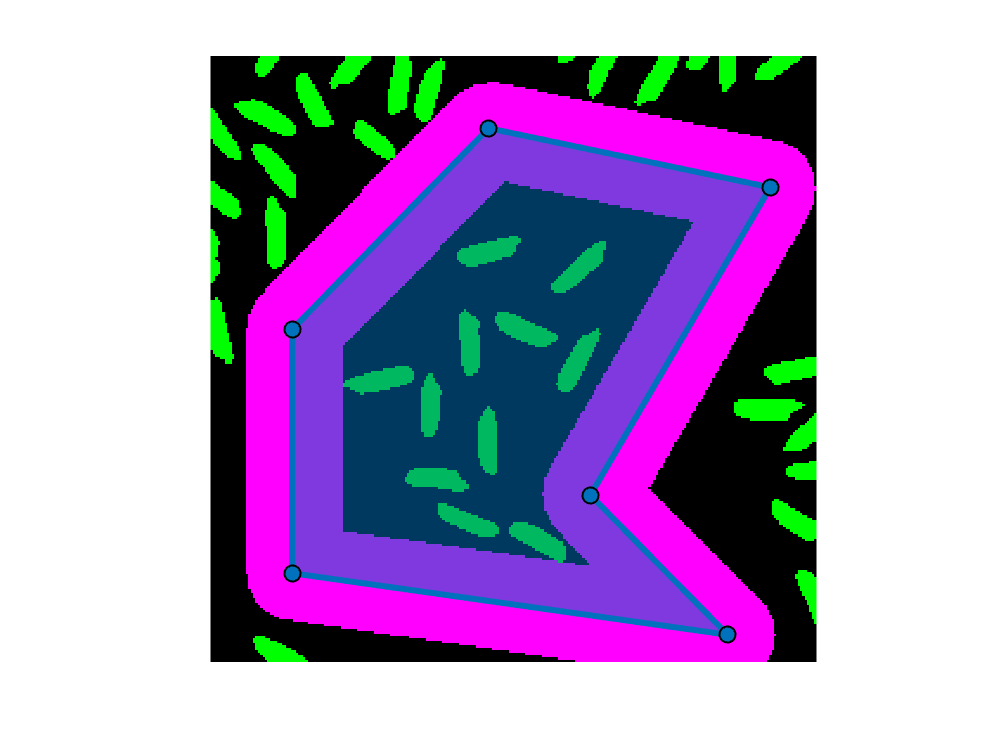This post demonstrated a number of MATLAB and toolbox functions and operators that work well together:
• Logical operators on binary images to compute things like "in this image or mask but not in this other one"
• imreconstruct to reconstruct original binary image shapes from partial ones
• bwdist to compute the distance transform, which tells you how far away every image pixel is from some binary image mask
• Relational operators to form masks from distance transform images
Have fun with these.
And Happy New Year!
function showpoly
% Helper function
xy = [ ...
118 31
35 116
35 219
219 245
161 186
237 56];

drawpolygon(Position = xy, FaceAlpha = 0.5);
end
|